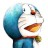# 杰拉斯的博客

## [ACM_ZJUT_1299]幸运妈妈杰拉斯 | 时间：2012-04-11, Wed | 24,070 views

### 幸运妈妈

Time Limit:1000MS Memory Limit:32768K

```
5
4
16
27
0
```

#### Sample Output

```0 0
2 2
2 4
3 3SourceZJUT1299这道题用了两种方法来做，都是Wrong Answer，不知为何，还请各位大牛指教！第一种，若XK = N，则K = logXN = lg(N) / lg(X)，那么通过判断K是否为整数来确定N能否写为N的K次方：#include<iostream>
#include<cmath>
#include<float.h>
int main(){
int n;
while(scanf("%d", &n) != EOF && n){
int X = 0, K = 0;
if(n == 1){
X = 1;
K = 2;
}
else
for(int i = 2; i <= sqrt((float)n); ++i){
double tmp = log((float)n) / log((float)i);
if(fabs(tmp - (int)tmp) <= FLT_EPSILON){	//判断tmp是否为整数
K = tmp;
X = i;
break;
}
//printf("%d\n", tmp);
}
printf("%d %d\n", X, K);
}
return 0;
}

#include<cmath>
#include<map>
using namespace std;
struct s{
int X;
int K;
};
int main(){
int n = 10000;
map<int, s> m;
m.X = 1;
m.K = 2;
for(int i = 2; i <= 100; ++i){
for(int j = 2; j < 15; ++j){
int num = pow((float)i, j);
if(num > 10000 || (m[num].X > 0 && m[num].X < i)){
break;
}
m[num].X = i;
m[num].K = j;
}
}
while(scanf("%d", &n) != EOF && n){
printf("%d %d\n", m[n].X, m[n].K);
}
return 0;
}

#include<cmath>
#include<map>
using namespace std;
struct s{
int X;
int K;
};
int main(){
int n = 10000;
map<int, s> m;
for(int i = 99; i >= 2; --i){
for(int j = 2; j < 15; ++j){
int num = pow((float)i, j);
if(num > 10000 || (m[num].X > 0 && m[num].K < j)){
continue;
}
m[num].X = i;
m[num].K = j;
}
}
while(scanf("%d", &n) != EOF && n){
printf("%d %d\n", m[n].X, m[n].K);
}
return 0;
}

using namespace std;
struct s{
int x,y;
} ;
int main(){
s a;
for(int k=1;k<=9999;k++)
{
a[k].x=0;a[k].y=0;
}
for(int i=2; i<100; i++){
int t=i*i;
for(int j=2; t<10000; j++,t*=i)
a[t].x=i, a[t].y=j;
}
for(int n; cin>>n && n; )
cout<<a[n].x<<" "<<a[n].y<<"\n";
}
如需转载请注明出处：杰拉斯的博客
相关文章

[ACM_Tyvj_1011]传纸条（双线程动态规划解法）
[ACM_NYOJ_19]擅长排列的小明
[ACM_NYOJ_47]过河问题
[ACM_NYOJ_27]水池数目
[ACM_NYOJ_8]一种排序

```

#### 5 条评论 »

1.maxwell

这应该是 K = logX N = lg(N) / lg(X) 被你误导了。。。

2.maxwell

第一种，四舍五入不对，左边 double 型，小数部分不一般为零；右边 int 型,运算时自动转换为 double 型，小数部分为零。两者必然不等。
判断两个 double 型相等一般用 fabs( d1 - d2 ) <= FLT_EPSILON ，而不是直接用 operator ==
（FLT_EPSILON 是 浮点运算相关常量，位于 float.h (ANSI C 头文件，具体值在不同编译器略有差别，也可以自己定义，一般取 1E-3~1E-5 就差不多了，看具体情况 )）
所以要判断 K == lg(N) / lg(X) 可以用
fabs( K - log(N)/log(X) ) <= FLT_EPSILON ，
我觉得写 ( K * log(X) - log(N) ) <= FLT_EPSILON 更好，一方面乘法效率比除法高，另外误差也更小。

改改改。。。
按你原来的思路，14行的 if 条件可以写 fabs( tmp - (int)(tmp + 0.5) ) <= FLT_EPSILON 试试
个人认为，第二种没什么必要

1.Chang

1.maxwell
2.maxwell